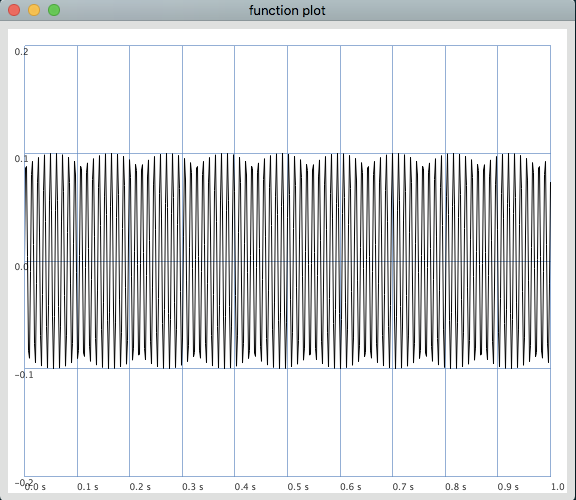# Indexing into a wavetable with Phasor()

Hello,

I would like to use `Phasor()` to drive the playback of a table that I create at audio rate.
I think I may be on the right track, but would appreciate help. What I notice is that when I attempt to load table `w` into b, `b`'s plot looks different than I would anticipate. `w` on the other hand looks correct.

Thanks for any thoughts; just getting back to SC after a long hiatus.

``````(
{ var b, w, idx;
b = Buffer.alloc(s, 512, 1);
w = Wavetable.sineFill(512, 1.0/);
w.plot;

//leaving out until b.plot looks like w.plot
//idx = Phasor.ar(Impulse.ar(1), 1, start:0, end:b.numFrames);
//BufRd.ar(1, b.bufnum, idx, 1, 2);
}.play;
)``````

Wavetables are in a special format to be used by wavetable oscillators (Osc, VOsc, Shaper, etc). You can use Signal.sineFill for BufRd

From Wavetable documentation:

This strange format is not a standard linear interpolation (integer + frac), but for (integer part -1) and (1+frac)) due to some efficient maths for integer to float conversion in the underlying C code.

1 Like

Short answer: Use Signal, not Wavetable, for this.

Why? As hemiketal said, Wavetable is a special format reserved for Osc (or OscN, COsc, VOsc, VOsc3) and Shaper. It does not just give you the sample values. If you’re using Phasor and BufRd, Wavetable is inappropriate. You need just the sample values, which will be provided by Signal.

``````w = Signal.sineFill(512, 1.0 / );

w.plot;

b = Buffer.alloc(s, 512, 1);

b.plot;

(
a = {
var idx = Phasor.ar(Impulse.ar(1), 1, start:0, end:b.numFrames);
BufRd.ar(1, b.bufnum, idx, 1, 2) * 0.1;
}.play;
)

a.release;
b.free;
``````

There are a couple more gotchas.

1. `Buffer.alloc` and `loadCollection` are language operations. They do cause things to happen on the server, but they can be triggered only from the language. It isn’t a good idea to write them into a synthesis function. (In the examples in this post, I’m moving buffer preparation outside of the synthesis function.)

2. Documentation is not clear on this point, but a Wavetable will be twice the size of the number of samples requested.

``````w = Wavetable.sineFill(512, 1.0/);

w.size;  // 1024 (!)
``````

So when you try to load this into a 512-sample buffer, you’re losing the last half of the wavetable. (So that’s two things that would make `b.plot` look different – wavetable format, and keeping only half the data.) This problem disappears by using Signal.

If you really want to use Wavetable, it would look like this – just like SinOsc, you can drive a wavetable oscillator by specifying 0 frequency and calculating phase yourself.

``````w = Wavetable.sineFill(512, 1.0/);

// note that this will allocate 1024 samples
// by checking w.size internally
// so that's one less mistake you can make

// at this point, b.plot will look funny, but that's OK...

(
a = {
var freq = 440;
// for this usage, phase must be in radians
var phase = Phasor.ar(0, (2pi * SampleDur.ir) * freq, 0, 2pi, 0);
(Osc.ar(b, 0, phase) * 0.1).dup
}.play;
)

// Osc makes a clean sine tone ;->

a.release;
b.free;
``````

hjh

1 Like

Thank you for the thorough explanation, @jamshark70 .
This cleared up a lot of issues I was having, and sets me straight on some important points!
I will try to brush up on the key tenets of lang vs. server, and reread relevant docs.

Have a good one!

I may be mistaken, but the Signal example provided above does not produce the desired outcome. If I plot 1 second of the function, it does not yield the expected cycle:

``````w = Signal.sineFill(512, 1.0 / );
w.plot;
b = Buffer.alloc(s, 512, 1);
b.plot;

(
a = {
var idx = Phasor.ar(Impulse.ar(1), 1, start:0, end:b.numFrames);
BufRd.ar(1, b.bufnum, idx, 1, 2) * 0.1;
}.plot(1);
)
``````

produces this:If you want to use this as you would an oscillator, I believe the rate of the Phasor must be a ratio of the buffer size to the sample rate then scaled to the desired frequency, and does not require an Impulse to trigger it:

``````(
~sineArray = Signal.sineFill(512, [1.0]);
)

(
{
~freq = 1;
~index = Phasor.ar(0, ~sineBuf.numFrames/SampleRate.ir * ~freq, 0, BufFrames.kr(~sineBuf));
BufRd.ar(1, ~sineBuf, ~index);
}.plot(1);
)
``````

New users can only post one image, so I’ll let you test for yourself.

Best,
w.

1 Like